### Factorisation Class 8th Mathematics AP Board Solution

##### Question 1.Find the errors and correct the following mathematical sentences3(x – 9) = 3x – 9Answer:If LHS is3(x – 9)Then RHS would be⇒ 3(x – 9)= 3 × x – 3 × 9= 3x – 27The error is 27 instead of 9Hence 3(x – 9) = 3x – 27Question 2.Find the errors and correct the following mathematical sentencesx(3x + 2) = 3x2 + 2Answer:If LHS isx(3x + 2)Then RHS would be⇒ x(3x + 2)= 3 × x × x – 2 × x= 3x2 – 2xThe error is 2x instead of 2Hence x(3x + 2) = 3x2 + 2xQuestion 3.Find the errors and correct the following mathematical sentences2x + 3x = 5x2Answer:If LHS is2x + 3xThen RHS would be⇒ 2x + 3x= x(2 + 3)= 5xThe error is 5x instead of 5x2Hence 2x + 3x = 5xQuestion 4.Find the errors and correct the following mathematical sentences2x + x + 3x = 5xAnswer:If LHS is2x + x + 3x = 5xThen RHS would be⇒ 2x + x + 3x= x(2 + 1 + 3)= 6xThe error is 6x instead of 5xHence 2x + x + 3x = 6xQuestion 5.Find the errors and correct the following mathematical sentences4p + 3p + 2p + p – 9p = 0Answer:If LHS is4p + 3p + 2p + p – 9pThen RHS would be⇒ 4p + 3p + 2p + p – 9p= p(4 + 3 + 2 + 1–9)= p(10–9)= pThe error is p instead of 0Hence 4p + 3p + 2p + p–9p = pQuestion 6.Find the errors and correct the following mathematical sentences3x + 2y = 6xyAnswer:If RHS is6xyThen LHS would be⇒ 6xy= 2 × 3 × x × y= 3 × x × 2 × y= 3x × 2yThe error is sign of multiplication instead of sign of additionHence 3x × 2y = 6xyQuestion 7.Find the errors and correct the following mathematical sentences(3x)2 + 4x + 7 = 3x2 + 4x + 7Answer:If LHS is(3x)2 + 4x + 7Then RHS would be⇒ (3x)2 + 4x + 7= 32 × x2 + 4x + 7= 9x2 + 4x + 7The error is 9x2 instead of 3x2Hence (3x)2 + 4x + 7 = 9x2 + 4x + 7Question 8.Find the errors and correct the following mathematical sentences(2x)2 + 5x = 4x + 5x = 9xAnswer:If LHS is(2x)2 + 5xThen RHS would be⇒ (2x)2 + 5x= 22 × x2 + 5x= 4x2 + 5xThe error is 4x2 instead of 4xHence (2x)2 + 5x = 4x2 + 5xQuestion 9.Find the errors and correct the following mathematical sentences(2a + 3)2 = 2a2 + 6a + 9Answer:If LHS is(2a + 3)2Then RHS would be⇒ (2a + 3)2= (2a)2 + 32 + 2 × 2a × 3= 4a2 + 9 + 12a= 4a2 + 12a + 9The error is 4a2 instead of 2a2 and 12a instead of 6aHence = (2a + 3)2 = 4a2 + 9 + 12aQuestion 10.Find the errors and correct the following mathematical sentencesSubstitute x = – 3 in(a) x2 + 7x + 12 = (–3)2 + 7 (–3) + 12 = 9 + 4 + 12 = 25Answer:If LHS isx2 + 7x + 12Then RHS would be⇒ x2 + 7x + 12Putting x = (-3)= (–3)2 + 7 (–3) + 12= 9 + (-21) + 12= 21-21= 0The error is (-21) instead of 4 and end result 0 instead of 25Hence putting x = (-3) in x2 + 7x + 12 results to 0Question 11.Find the errors and correct the following mathematical sentencesSubstitute x = – 3 in(b) x2– 5x + 6 = (–3)2 –5 (–3) + 6 = 9 – 15 + 6 = 0Answer:If LHS isx2– 5x + 6Then RHS would be⇒ x2– 5x + 6Putting x = (-3)= (–3)2 –5 (–3) + 6= 9 + 15 + 6= 30The error is + 15 instead of (-15) and end results to 30 instead of 0Hence putting x = (-3) in x2– 5x + 6 results to 30Question 12.Find the errors and correct the following mathematical sentencesSubstitute x = – 3 in(c) x2 + 5x = (–3)2 + 5 (–3) + 6 = – 9 – 15 = –24Answer:If LHS isx2 + 5xThen RHS would be⇒ x2 + 5xPutting x = (-3)= (–3)2 + 5 (–3)= 9 + (-15)= -6The error is ( + 9) instead of (-9) and end results to (-6) instead of (-24)Hence putting x = (-3) in x2 + 5x results to (-6)Question 13.Find the errors and correct the following mathematical sentences(x – 4)2 = x2 – 16Answer:If LHS is(x – 4)2Then RHS would be⇒ (x – 4)2= (x)2 + 42 – 2 × x × 4= x2 + 16 – 8xThe error is x2 + 16 – 8x instead of x2 – 16Hence (x – 4)2 = x2 + 13 – 8xQuestion 14.Find the errors and correct the following mathematical sentences(x + 7)2 = x2 + 49Answer:If LHS is(x + 7)2Then RHS would be⇒ (x + 7)2= (x)2 + 72 + 2 × x × 7= x2 + 49 + 14The error is x2 + 14x + 49 instead of x2 + 49Hence (x + 7)2 = x2 + 14x + 49Question 15.Find the errors and correct the following mathematical sentences(3a + 4b) (a – b) = 3a2 – 4a2Answer:For getting in the equation(a2 – b2 ) = (a + b)(a-b)RHS would be3a2 – 4b2Then LHS would be⇒ 3a2 – 4b2= (3a – 4b)(3a + 4b)The error is (a – b) instead of (3a – 4b)3a2 – 4b2 instead of 3a2 – 4a2Hence 3a2 – 4b2 = (3a – 4b)(3a + 4b)Question 16.Find the errors and correct the following mathematical sentences(x + 4) (x + 2) = x2 + 8Answer:If LHS is(x + 4) (x + 2)Then RHS would be⇒ (x + 4) (x + 2)= x2 + 4 × x + 2 × x + 2 × 4= x2 + 4x + 2x + 8= x2 + 6x + 8The error is x2 + 6x + 8 instead of x2 + 8Hence (x + 4) (x + 2) = x2 + 6x + 8Question 17.Find the errors and correct the following mathematical sentences(x – 4) (x – 2) = x2 – 8Answer:If LHS is(x – 4) (x – 2)Then RHS would be⇒ (x – 4) (x – 2)= x2 – 4 × x – 2 × x + (-2) × (-4)= x2 – 4x – 2x + 8= x2 – 6x + 8The error is x2 – 6x + 8 instead of x2 – 8Hence (x – 4) (x – 2) = x2 – 6x + 8Question 18.Find the errors and correct the following mathematical sentences5x3 ÷ 5x3 = 0Answer:If LHS is5x3 ÷ 5x3Then RHS would be⇒ 5x3 ÷ 5x3=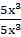= 1The error is1 instead of 0Hence 5x3 ÷ 5x3 = 1Question 19.Find the errors and correct the following mathematical sentences2x3 + 1 ÷ 2x3 = 1Answer:If LHS is(2x3 + 1) ÷ 2x3Then RHS would be⇒ (2x3 + 1) ÷ 2x3=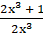=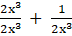=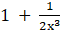The error isinstead of 1Hence (2x3 + 1) ÷ 2x3 =Question 20.Find the errors and correct the following mathematical sentences3x + 2 ÷ 3x =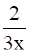Answer:If LHS is(3x + 2) ÷ 3xThen RHS would be⇒ (3x + 2) ÷ 3x=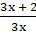=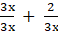=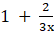The error isinstead of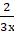Hence (3x + 2 )÷ 3x =Question 21.Find the errors and correct the following mathematical sentences3x + 5 ÷ 3 = 5Answer:If LHS isFor the complete and perfect divisionThere must be 3x instead of x(3x + 5)÷3xThen RHS would be⇒ (3x + 5)÷3x=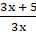=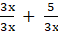=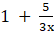The error isinstead of 5 and 3x instead of xHence = (3x + 5)÷3x =Question 22.Find the errors and correct the following mathematical sentences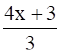= x + 1Answer:If LHS is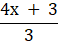Then RHS would be⇒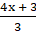=x +=x + 1The error isx + 1 instead of x + 1Hence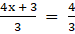x + 1

PDF FILE TO YOUR EMAIL IMMEDIATELY PURCHASE NOTES & PAPER SOLUTION. @ Rs. 50/- each (GST extra)

HINDI ENTIRE PAPER SOLUTION

MARATHI PAPER SOLUTION

SSC MATHS I PAPER SOLUTION

SSC MATHS II PAPER SOLUTION

SSC SCIENCE I PAPER SOLUTION

SSC SCIENCE II PAPER SOLUTION

SSC ENGLISH PAPER SOLUTION

SSC & HSC ENGLISH WRITING SKILL

HSC ACCOUNTS NOTES

HSC OCM NOTES

HSC ECONOMICS NOTES

HSC SECRETARIAL PRACTICE NOTES

# 2019 Board Paper Solution

HSC ENGLISH SET A 2019 21st February, 2019

HSC ENGLISH SET B 2019 21st February, 2019

HSC ENGLISH SET C 2019 21st February, 2019

HSC ENGLISH SET D 2019 21st February, 2019

SECRETARIAL PRACTICE (S.P) 2019 25th February, 2019

HSC XII PHYSICS 2019 25th February, 2019

CHEMISTRY XII HSC SOLUTION 27th, February, 2019

OCM PAPER SOLUTION 2019 27th, February, 2019

HSC MATHS PAPER SOLUTION COMMERCE, 2nd March, 2019

HSC MATHS PAPER SOLUTION SCIENCE 2nd, March, 2019

SSC ENGLISH STD 10 5TH MARCH, 2019.

HSC XII ACCOUNTS 2019 6th March, 2019

HSC XII BIOLOGY 2019 6TH March, 2019

HSC XII ECONOMICS 9Th March 2019

SSC Maths I March 2019 Solution 10th Standard11th, March, 2019

SSC MATHS II MARCH 2019 SOLUTION 10TH STD.13th March, 2019

SSC SCIENCE I MARCH 2019 SOLUTION 10TH STD. 15th March, 2019.

SSC SCIENCE II MARCH 2019 SOLUTION 10TH STD. 18th March, 2019.

SSC SOCIAL SCIENCE I MARCH 2019 SOLUTION20th March, 2019

SSC SOCIAL SCIENCE II MARCH 2019 SOLUTION, 22nd March, 2019

XII CBSE - BOARD - MARCH - 2019 ENGLISH - QP + SOLUTIONS, 2nd March, 2019

# HSCMaharashtraBoardPapers2020

(Std 12th English Medium)

HSC ECONOMICS MARCH 2020

HSC OCM MARCH 2020

HSC ACCOUNTS MARCH 2020

HSC S.P. MARCH 2020

HSC ENGLISH MARCH 2020

HSC HINDI MARCH 2020

HSC MARATHI MARCH 2020

HSC MATHS MARCH 2020

# SSCMaharashtraBoardPapers2020

(Std 10th English Medium)

English MARCH 2020

HindI MARCH 2020

Hindi (Composite) MARCH 2020

Marathi MARCH 2020

Mathematics (Paper 1) MARCH 2020

Mathematics (Paper 2) MARCH 2020

Sanskrit MARCH 2020

Sanskrit (Composite) MARCH 2020

Science (Paper 1) MARCH 2020

Science (Paper 2)

Geography Model Set 1 2020-2021

MUST REMEMBER THINGS on the day of Exam

Are you prepared? for English Grammar in Board Exam.

Paper Presentation In Board Exam

How to Score Good Marks in SSC Board Exams

Tips To Score More Than 90% Marks In 12th Board Exam

How to write English exams?

How to prepare for board exam when less time is left

How to memorise what you learn for board exam

No. 1 Simple Hack, you can try out, in preparing for Board Exam

How to Study for CBSE Class 10 Board Exams Subject Wise Tips?

JEE Main 2020 Registration Process – Exam Pattern & Important Dates

NEET UG 2020 Registration Process Exam Pattern & Important Dates

How can One Prepare for two Competitive Exams at the same time?

8 Proven Tips to Handle Anxiety before Exams!